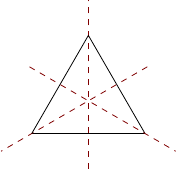0Introduction

IA Groups0 Introduction
Group theory is an example of algebra. In pure mathematics, algebra (usually)
does not refer to the boring mindless manipulation of symbols. Instead, in
algebra, we have some set of objects with some operations on them. For example,
we can take the integers with addition as the operation. However, in algebra, we
allow any set and any operations, not just numbers.
Of course, such a definition is too broad to be helpful. We categorize algebraic
structures into different types. In this course, we will study a particular kind of
structures, groups. In the IB Groups, Rings and Modules course, we will study
rings and modules as well.
These different kinds of structures are defined by certain axioms. The group
axioms will say that the operation must follow certain rules, and any set and
operation that satisfies these rules will be considered to form a group. We will
then have a different set of axioms for rings, modules etc.
As mentioned above, the most familiar kinds of algebraic structures are
number systems such as integers and rational numbers. The focus of group
theory, however, is not on things that resemble “numbers”. Instead, it is the
study of symmetries.
First of all, what is a symmetry? We are all familiar with, say, the symmetries
of an (equilateral) triangle (we will always assume the triangle is equilateral). We
rotate a triangle by 120
, and we get the original triangle. We say that rotating
by 120
is a symmetry of a triangle. In general, a symmetry is something we do
to an object that leaves the object intact.
Of course, we don’t require that the symmetry leaves everything intact.
Otherwise, we would only be allowed to do nothing. Instead, we require certain
important things to be intact. For example, when considering the symmetries
of a triangle, we only care about how the resultant object looks, but don’t care
about where the individual vertices went.
In the case of the triangle, we have six symmetries: three rotations (rotation
by 0
, 120
and 240
), and three reflections along the axes below:
These six together form the underlying set of the group of symmetries. A more
sophisticated example is the symmetries of
R
3
. We define these as operations on
R
3
that leave distances between points unchanged. These include translations,
rotations, reflections, and combinations of these.
So what is the operation? This operation combines two symmetries to give a
new symmetry. The natural thing to do is to do the symmetry one after another.
For example, if we combine the two 120
rotations, we get a 240
rotation.
Now we are studying algebra, not geometry. So to define the group, we
abstract away the triangle. Instead, we define the group to be six objects, say
{e, r, r
2
, s, rs, r
2
s}
, with rules defining how we combine two elements to get a
third. Officially, we do not mention the triangle at all when defining the group.
We can now come up with the group axioms. What rules should the set of
symmetries obey? First of all, we must have a “do nothing” symmetry. We call
this the identity element. When we compose the identity with another symmetry,
the other symmetry is unchanged.
Secondly, given a symmetry, we can do the reverse symmetry. So for any
element, there is an inverse element that, when combined with the original, gives
the identity.
Finally, given three symmetries, we can combine them, one after another. If
we denote the operation of the group as
, then if we have three symmetries,
x, y, z
, we should be able to form
x y z
. If we want to define it in terms of
the binary operation
, we can define it as (
x y
)
z
, where we first combine
the first two symmetries, then combine the result with the third. Alternatively,
we can also define it as
x
(
y z
). Intuitively, these two should give the same
result, since both are applying
x
after
y
after
z
. Hence we have the third rule
x (y z) = (x y) z.
Now a group is any set with an operation that satisfies the three rules above.
In group theory, the objective is to study the properties of groups just assuming
these three axioms. It turns out that there is a lot we can talk about.